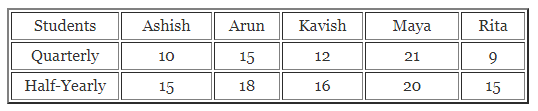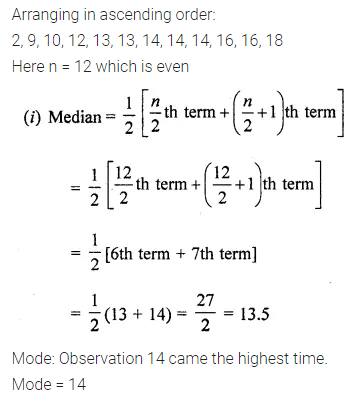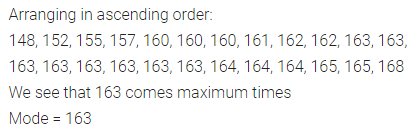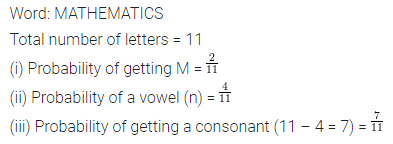# ML Aggarwal Class 7 Solutions for ICSE Maths Chapter 17 Data Handling Check Your Progress

## ML Aggarwal Class 7 Solutions for ICSE Maths Chapter 17 Data Handling Check Your Progress

Question 1.
State whether the following statement is true or false. Justify your answer:
“The median is always one of the numbers in data.”
Solution:Question 2.
Marks obtained by five students of class VII in quarterly and half-yearly examination in Mathematics (out of 25) are given below:Represent the above data by a bar graph and answer the following questions:
(ii) Who has not done better?
Solution:Question 3.
Find the mean of the factors of 12.
Solution:Question 4.
Find the median and the mode of the following data:
2, 14, 16, 12, 13, 14, 16, 13, 10, 14, 18, 9
Solution:Question 5.
Heights (in cm) of 25 students are given below:
168, 165, 163, 160, 163, 161, 162, 164, 163, 162, 164, 163, 160, 163, 157, 165, 148, 163, 164, 160, 165, 163, 152, 155, 163.
What is the mode of their heights?
Solution:Question 6.
A die is thrown, what is the probability of getting an odd prime number?
Solution:Question 7.
A letter is chosen at random from the letters of the word MATHEMATICS, what is the probability of getting
(i) letter M
(ii) a vowel
(iii) a consonant?
Solution: